## Use the degree and leading coefficient to describe end behavior of polynomial functions

### Identify polynomial functions

An oil pipeline bursts in the Gulf of Mexico, causing an oil slick in a roughly circular shape. The slick is currently 24 miles in radius, but that radius is increasing by 8 miles each week. We want to write a formula for the area covered by the oil slick by combining two functions. The radius r of the spill depends on the number of weeks w that have passed. This relationship is linear.

$r\left(w\right)=24+8w$

We can combine this with the formula for the area A of a circle.

$A\left(r\right)=\pi {r}^{2}$

Composing these functions gives a formula for the area in terms of weeks.

$\begin{cases}A\left(w\right)=A\left(r\left(w\right)\right)\\ =A\left(24+8w\right)\\ =\pi {\left(24+8w\right)}^{2}\end{cases}$

Multiplying gives the formula.

$A\left(w\right)=576\pi +384\pi w+64\pi {w}^{2}$

This formula is an example of a polynomial function. A polynomial function consists of either zero or the sum of a finite number of non-zero terms, each of which is a product of a number, called the coefficient of the term, and a variable raised to a non-negative integer power.

### A General Note: Polynomial Functions

Let n be a non-negative integer. A polynomial function is a function that can be written in the form

$f\left(x\right)={a}_{n}{x}^{n}+\dots+{a}_{2}{x}^{2}+{a}_{1}x+{a}_{0}$

This is called the general form of a polynomial function. Each ${a}_{i}$ is a coefficient and can be any real number. Each product ${a}_{i}{x}^{i}$ is a term of a polynomial function.

### Example 4: Identifying Polynomial Functions

Which of the following are polynomial functions?

$\begin{cases}f\left(x\right)=2{x}^{3}\cdot 3x+4\hfill \\ g\left(x\right)=-x\left({x}^{2}-4\right)\hfill \\ h\left(x\right)=5\sqrt{x}+2\hfill \end{cases}$

### Solution

The first two functions are examples of polynomial functions because they can be written in the form $f\left(x\right)={a}_{n}{x}^{n}+\dots+{a}_{2}{x}^{2}+{a}_{1}x+{a}_{0},$ where the powers are non-negative integers and the coefficients are real numbers.

$f\left(x\right)$
can be written as $f\left(x\right)=6{x}^{4}+4.$

$g\left(x\right)$
can be written as $g\left(x\right)=-{x}^{3}+4x.$

$h\left(x\right)$
cannot be written in this form and is therefore not a polynomial function.

## Define the degree and leading coefficient of a polynomial function

Because of the form of a polynomial function, we can see an infinite variety in the number of terms and the power of the variable. Although the order of the terms in the polynomial function is not important for performing operations, we typically arrange the terms in descending order of power, or in general form. The degree of the polynomial is the highest power of the variable that occurs in the polynomial; it is the power of the first variable if the function is in general form. The leading term is the term containing the highest power of the variable, or the term with the highest degree. The leading coefficient is the coefficient of the leading term.

### A General Note: Terminology of Polynomial FunctionsFigure 6

We often rearrange polynomials so that the powers are descending.

When a polynomial is written in this way, we say that it is in general form.

### How To: Given a polynomial function, identify the degree and leading coefficient.

1. Find the highest power of x to determine the degree function.
2. Identify the term containing the highest power of x to find the leading term.
3. Identify the coefficient of the leading term.

### Example 5: Identifying the Degree and Leading Coefficient of a Polynomial Function

Identify the degree, leading term, and leading coefficient of the following polynomial functions.

$\begin{cases} f\left(x\right)=3+2{x}^{2}-4{x}^{3} \\ g\left(t\right)=5{t}^{5}-2{t}^{3}+7t\\ h\left(p\right)=6p-{p}^{3}-2\end{cases}$

### Solution

For the function $f\left(x\right),$ the highest power of x is 3, so the degree is 3. The leading term is the term containing that degree, $-4{x}^{3}.$ The leading coefficient is the coefficient of that term, –4.

For the function $g\left(t\right),$ the highest power of t is 5, so the degree is 5. The leading term is the term containing that degree, $5{t}^{5}.$ The leading coefficient is the coefficient of that term, 5.

For the function $h\left(p\right),$ the highest power of p is 3, so the degree is 3. The leading term is the term containing that degree, $-{p}^{3};$ the leading coefficient is the coefficient of that term, –1.

### Try It 3

Identify the degree, leading term, and leading coefficient of the polynomial $f\left(x\right)=4{x}^{2}-{x}^{6}+2x - 6.$

Solution

## Identifying End Behavior of Polynomial Functions

Knowing the degree of a polynomial function is useful in helping us predict its end behavior. To determine its end behavior, look at the leading term of the polynomial function. Because the power of the leading term is the highest, that term will grow significantly faster than the other terms as x gets very large or very small, so its behavior will dominate the graph. For any polynomial, the end behavior of the polynomial will match the end behavior of the term of highest degree.

Polynomial Function Leading Term Graph of Polynomial Function
$f\left(x\right)=5{x}^{4}+2{x}^{3}-x - 4$ $5{x}^{4}$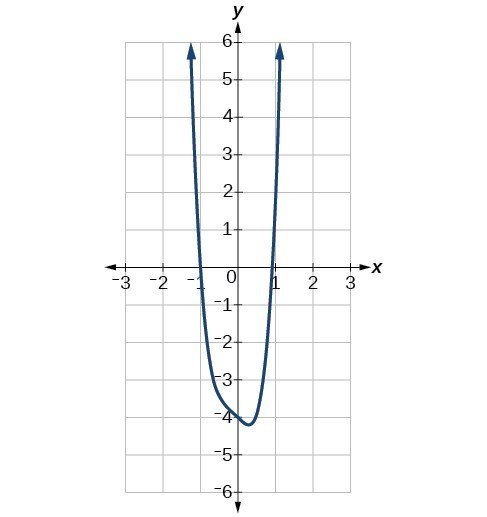$f\left(x\right)=-2{x}^{6}-{x}^{5}+3{x}^{4}+{x}^{3}$ $-2{x}^{6}$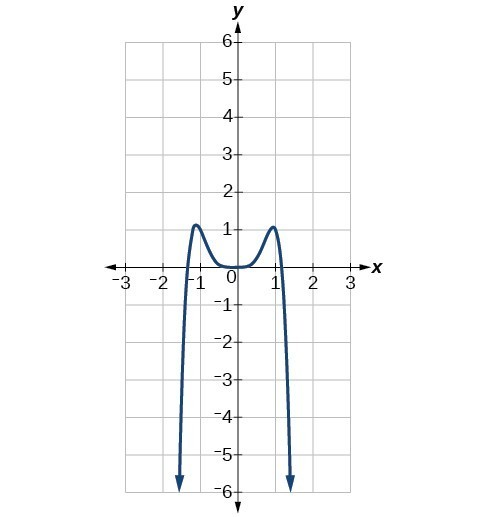$f\left(x\right)=3{x}^{5}-4{x}^{4}+2{x}^{2}+1$ $3{x}^{5}$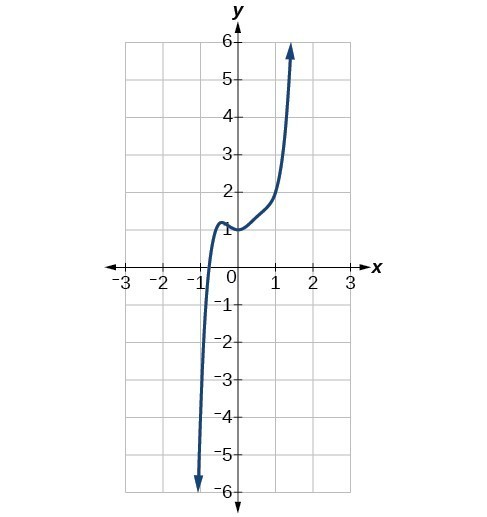$f\left(x\right)=-6{x}^{3}+7{x}^{2}+3x+1$ $-6{x}^{3}$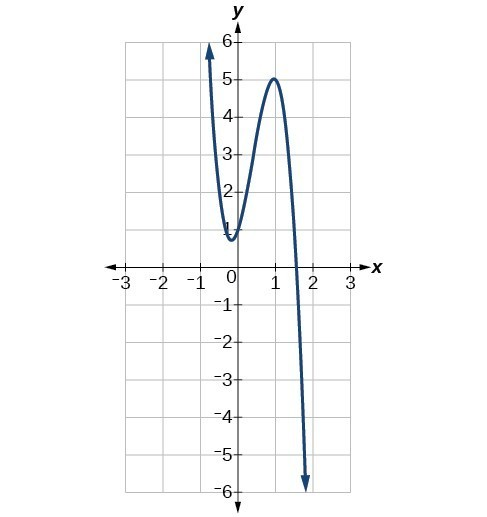### Example 6: Identifying End Behavior and Degree of a Polynomial Function

Describe the end behavior and determine a possible degree of the polynomial function in Figure 7.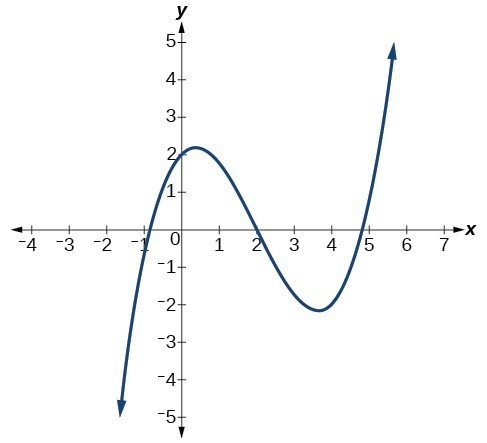Figure 7

### Solution

As the input values x get very large, the output values $f\left(x\right)$ increase without bound. As the input values x get very small, the output values $f\left(x\right)$ decrease without bound. We can describe the end behavior symbolically by writing

$\begin{cases}\text{as } x\to -\infty , f\left(x\right)\to -\infty \\ \text{as } x\to \infty , f\left(x\right)\to \infty \end{cases}$

In words, we could say that as x values approach infinity, the function values approach infinity, and as x values approach negative infinity, the function values approach negative infinity.

We can tell this graph has the shape of an odd degree power function that has not been reflected, so the degree of the polynomial creating this graph must be odd and the leading coefficient must be positive.

### Try It 4

Describe the end behavior, and determine a possible degree of the polynomial function in Figure 9.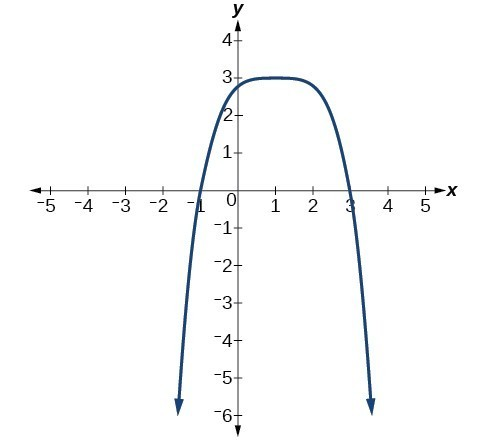Figure 9

Solution

### Example 7: Identifying End Behavior and Degree of a Polynomial Function

Given the function $f\left(x\right)=-3{x}^{2}\left(x - 1\right)\left(x+4\right),$ express the function as a polynomial in general form, and determine the leading term, degree, and end behavior of the function.

### Solution

Obtain the general form by expanding the given expression for $f\left(x\right).$

$\begin{cases} f\left(x\right)=-3{x}^{2}\left(x - 1\right)\left(x+4\right)\\ \hfill =-3{x}^{2}\left({x}^{2}+3x - 4\right)\\ \hfill=-3{x}^{4}-9{x}^{3}+12{x}^{2}\end{cases}$

The general form is $f\left(x\right)=-3{x}^{4}-9{x}^{3}+12{x}^{2}.$ The leading term is $-3{x}^{4};$ therefore, the degree of the polynomial is 4. The degree is even (4) and the leading coefficient is negative (–3), so the end behavior is

$\begin{cases}\text{as } x\to -\infty , f\left(x\right)\to -\infty \\ \text{as } x\to \infty , f\left(x\right)\to -\infty \end{cases}$

### Try It 5

Given the function $f\left(x\right)=0.2\left(x - 2\right)\left(x+1\right)\left(x - 5\right),$ express the function as a polynomial in general form and determine the leading term, degree, and end behavior of the function.

Solution